JOINProblem Statement
Contest: Asteroid Tracker
Problem: AsteroidTracker

# Background

In 2013, over a dozen large asteroids similar in size to the object that crashed into Russia in February 2013 passed near Earth. Near-Earth Object ("NEO") detection and characterization is a critical need for NASA, the US, and the world as a whole. NASA has been directed to develop capabilities to observe, track and characterize NEOs and other deep space objects that could pose a threat to the Earth. As a result, NASA is developing concepts for a highly capable deep space radar array consisting of sets of commercially available monolithic antennas.

NASA's Ka-Band Object Observation and Monitoring ("KaBOOM") project plans to use commercial 12 meter Ka-band radar dishes to build large arrays capable of simultaneously tracking many objects. This raises the possibility that the detection range for Earth-bound asteroids can be inexpensively extended and maintained. One of the challenges faced by NASA is determining the optimum selection of individual antennas within the array for a given track observation. This is a complex analysis and goes directly to development of the concept of operations and cost of operations (in terms of maintenance and total capacity required).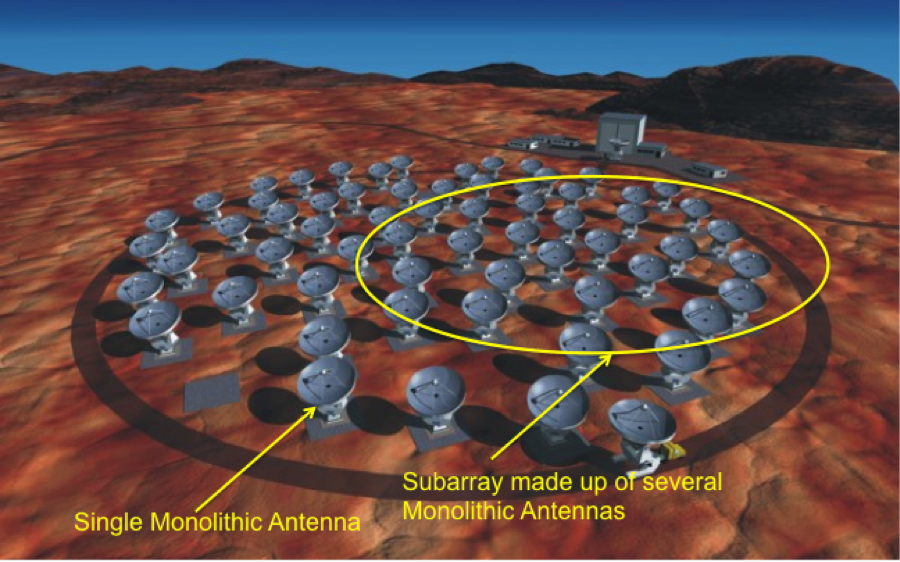Refer to the KaBOOM official minisite for more information.

# Problem specification

In this particular challenge, your task is to optimize the use of an array of radar dishes when tracking a number of NEOs over a time period. This tracking allows scientists to gather information from each object such as imagery and accurate trajectory. The goal is to maximize the science return over the set of objects in the given time period while also minimizing the energy consumption.

For each moment in the time period, you will need to specify which antennas should form a subarray, and which asteroid each subarray should target. You will also need to specify the transmitting power of each antenna. When you relocate an antenna to a new target, it rapidly slews towards the target with constant angular velocity. When the antenna reaches the new target, it will continue to turn at a much lower rate to continue to keep the target in its beam as it tracks. Also, when the antenna reaches the new target, it will immediately join any existing subarray targeting that asteroid, and the output signal power from the subarray will be updated with the contribution from the new antenna. While an antenna is slewing to a new target, it does not emit any signal, regardless of the specified output power. Subarrays also receive the reflected signal back from the asteroid they are targeting. It takes some time for the signal to travel back and forth to the asteroid, so it is not necessarily the same antennas transmitting the signal that receives it. The round trip delay time is two times the distance to the asteroid divided by the speed of light. The returning signal does not come for free; it also has some noise that includes background noise, and noise that is induced from other antennas off-axis radiation that hits signal-receiving equipment. The rate of the information gained for an asteroid at any moment is calculated as the power signal-to-noise ratio in logarithmic decibel scale. All antennas in a subarray receives the reflected signal automatically without you needing to specify anything. Antennas can transmit and receive signals simultaneously.

The simulation updates in discrete events. There is no explicit timer and the simulation is constant between events. You will need to implement a function that returns your next command. You can return two kinds of commands:

• change transmitting power to p for antenna j at time t
• start to relocate antenna j to asteroid i at time t
Changing transmitting power is instantaneous, but relocating an antenna takes some time. If you decide to interrupt the relocation, the antenna will be partially moved. Between each pair of consecutive events, a relocating antenna rotates with constant angular velocity towards its target. If the targeted asteroid happens to move during relocation, the antenna will start rotating towards the new target position until it finally catches up. Once a relocating antenna reaches its target, it will stay in sync with the target. You can relocate multiple antennas simultaneously. You can turn an antenna off by relocating to index -1.

Your command will be added to a list of events that will eventually happen. The simulation will then proceed and execute event after event until one of two things happens:

• a new asteroid appears
• your command starts to be executed
If a new asteroid appears, your program will be informed, and you will be requested for a new command. Your previous command will be ignored in this case so that you have the ability to change your mind based on the new information. Otherwise, if no new asteroid appears before your specified command, the command starts to be executed, and you will be requested for a new command, and the process repeats. Besides your commands and new asteroid appearances, there are three other kinds of events:
• asteroid i changes position to u at time t
• antenna j is done relocating at time t
• the returned signal from asteroid i changes at time t
These events are deterministic and generated automatically by the simulation. Your program will not be notified about these events, and they will not interrupt your commands. Asteroid positions are constant between change position events.

Asteroids may go below the horizon. The positions of the asteroids are given relative to the antenna array. The z-axis points towards the sky in the positive direction. This means that asteroid positions with negative z-values are below the horizon and not visible. If an antenna is tracking an asteroid that is below the horizon it automatically turns idle and relocates to the place the asteroid will appear over the horizon in the future.

Your score will be integrated over the time period of the simulation and is determined by the energy you use and the information you gain about the asteroids.

## Effective signal power return

Several factors influcene the effective signal power return. These are:

• distance to the asteroid
• number of antennas transmitting, and their individual transmitting power
• number of antennas receiving the signal
• reflectivity and cross section area of asteroid
• peak gain: power transmitted in peak direction compared to an isotropic source
• how accurate the trajectory information of the object is

### Signal power loss due to distance

When a signal propagates through space it spreads over an increasingly large surface, so the power per unit area decreases quadratically with distance. Once the signal reaches the target and is reflected back, the process begins again, only now the "transmitting" power is only as much as was available at the target. So the total power loss for the round trip is proportional to the fourth power of the distance.

### Beamforming

The combined signal power in a subarray is greater than the sum of their individual effects. This is because the combined gain in the main beam direction grows quadratically with the number of antennas. The gain is multiplied both when transmitting and receiving the signal, so the round trip signal strength is proportional to the fourth power of the number of antennas.

### Reflection multiplier

Each asteroid i has a reflectivityMultiplieri for the signal strength. This reflection multiplier is constant for each asteroid througout the simulation and is multiplied with the signal power. This value takes into account properties such as asteroid cross section area and asteroid reflectivity.

### Peak gain

All antennas are identical and have the same characteristics in this contest. The value peakGain is multiplied with the signal power. This value represents the amount of power transmitted in the direction of peak radiation to that of an isotropic source.

### Accurate trajectory information

The complete trajectory of an asteroid will be given to you the first moment the asteroid appears. This trajectory is not very accurate, but can be used for calculating values such as distance and when the asteroid goes beyond the horizon etc. However, when aiming the antennas towards an asteroid, very accurate trajectory information is needed because the subarray has a very focused beam. There will be some loss in the returned signal power due to miss-targeting and this is represented by the trajectory information factor Ti,t for asteroid i at time t. This value change over time depending on how much you track the object and has an upper bound of 1. The formula for Ti,t will be given later.

### Formula

The effective signal power return wi,t for an asteroid i at time t is calculated in the following way: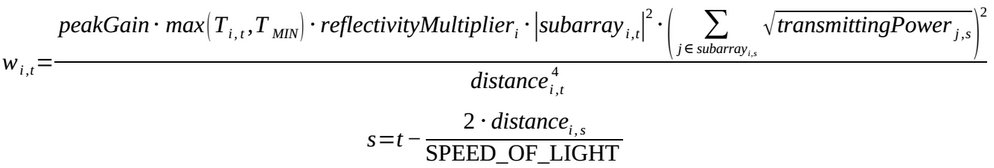where s is the time the signal was sent, T_MIN represents the capability to aim the antennas based on general information (possibly received from other sources), subarrayi,s are the antennas targeting the asteroid at time s, |subarrayi,t| is the number of antennas targeting the asteroid at time t, transmittingPowerj,s is the transmitting power of antenna j at time s, and distancei,t is the distance to the asteroid at time t. Note that distancei,s, the distance the moment the signal is sent, is used for calculating t, while distancei,t, the distance the moment the signal is received, is used for calculating wi,t.

## Noise

The returned signal is associated with noise n that includes background noise and induced noise on each of the receiving antennas. Each source of noise is uncorrelated, so their power sum simply summate. The background noise is constant in this contest, and is added for each receiving antenna.

### Induced noise

The antennas in this contest have parabolic reflectors that are designed to produce very focused beams in the direction of the dish axis, but some of the power will be radiated off-axis. This off-axis radiation will hit the other antennas signal-receiving equipment and induce noise into the measurements. The induced noise for a receiving antenna is the sum of the off-axis radiation power from all other antennas at the location of the receiving antenna. The off-axis radiation power is a function of the distance from the antenna, and the angle from the peak direction. The radiation power decreases quadratically with distance. The gain for different angles from peak direction will be given as a numerical array. The first entry in the array represents the angle 0°, i.e. peak direction, and the last entry represents the angle 180°, i.e. the opposite of peak direction. The other entries represents angles that are spread out linearly over the array. Two such arrays will be given that are generated for two different distances: the minimum and maximum distance between any pair of antennas in the testcase. The gain for distances between these values are calculated by interpolating these arrays linearly. Similarly, the gain for angles between entries in the array should also be interpolated linearly. The peak direction of the receiving antenna does not affect the induced noise. The induced noise for a receiving antenna j at time t is calculated in the following way: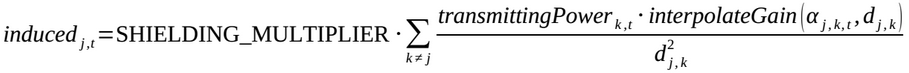where SHIELDING_MULTIPLIER represents the electromagnetic shielding of the signal-receiving equipment, αj,k,t is the angle between the peak direction of antenna k and the direction towards antenna j at time t, dj,k is the distance between antenna j and antenna k, and the function interpolateGain interpolates the gain bilinearly as described above.

The total noise ni,t associated with a signal received from asteroid i at time t is calculated in the following way: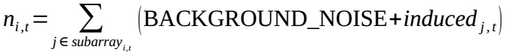where subarrayi,t are the antennas targeting the asteroid at time t.

## Information rate

The rate of the information gained for an asteroid i at time t is calculated as the power signal-to-noise ratio in logarithmic decibel scale: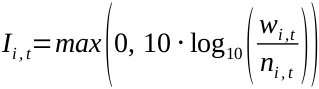## Energy consumption

The antennas consume energy when they are operating. The power consumption for an antenna is the transmitting power when tracking a target, or the power RELOCATE_POWER necessary to slew the antenna dish when relocating to a new target. The power required to rotate the dish while following a target is ignored in this contest. The total energy consumption is the integral of the antenna powers over the simulation time.

## Constraints

If you violate any of the following constraints your score will be zero for the test case. Constraints are only checked when time passes between events, they are not checked between events scheduled for the exact same time. This means that you can safely return a command with the exact same time t as an asteroid position change, i.e. you don't need to return a time t ± eps to ensure that your command is executed before/after the asteroid position change to avoid breaking a constraint.

### Near-field constraint

Besides inducing noise in other antennas signal-receiving equipment, the radiation emitted from antennas might actually cause physical damage to other antennas if the peak direction of the beam comes too close to another antenna. This is modeled as a geometrical constraint, where the ray in the peak direction of the beam is not allowed to come closer than a certain safety radius ANTENNA_SAFE_RADIUS to another antenna. This constraint only applies when the antenna is transmitting. An antenna is transmitting if it satisfies the following conditions:

• it is on, i.e. targeted asteroid index ≠ -1
• transmitting power > 0
• it is not currently relocating
• the targeted asteroid is above the horizon

### Target proximity constraint

Subarrays are not allowed to simultaneously target objects where the synthesized beam from one subarray intersects another targeted object. A subarray with few elements will have a wider beam width. The minimum allowed angle (in radians) between two tracked targets a and b is: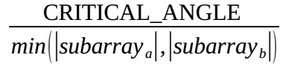where CRITICAL_ANGLE is a constant.

### Maximum transmitting power

The antennas have an individual maximum transmitting power of MAX_TRANSMITTING_POWER. If you try setting the transmitting power above this you break the constraint.

## Score

The score is the sum of the science return over the set of asteroids minus the total energy used. The science return for an asteroid is the trajectory knowledge score plus the image knowledge score. These knowledge score values are calculated as functions of the information rate over time.

### Image knowledge score

The image knowledge score for an asteroid i at time t is calculated in the following way: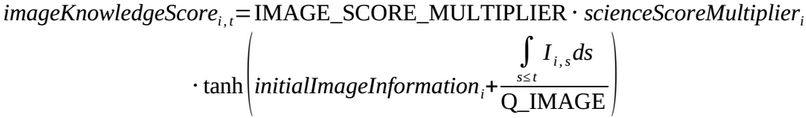where IMAGE_SCORE_MULTIPLIER is a constant that represents the importance of image information, scienceScoreMultiplieri is an asteroid specific constant that represents the importance of that asteroid, initialImageInformationi is the initial image information about the asteroid at the first appearance, Q_IMAGE is a constant that determines how much information is needed to get an image of good quality, and Ii,s is the information rate for asteroid i at time s.

### Trajectory knowledge score

The trajectory information of an asteroid decays over time. The trajectory information Ti,t for an asteroid i at time t is calculated in the following way: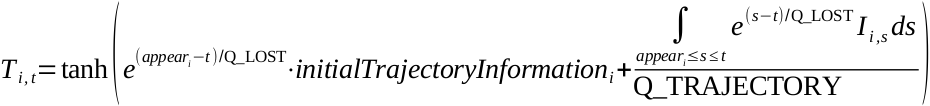where appeari is the time of the first appearance of the asteroid, initialTrajectoryInformationi is the initial trajectory information about the asteroid at the first appearance, Q_TRAJECTORY is a constant that determines how much information is needed to get accurate trajectory information, and Q_LOST is a constant that determines how slow we lose track of objects.

The trajectory knowledge score for an asteroid i at time t is calculated in the following way: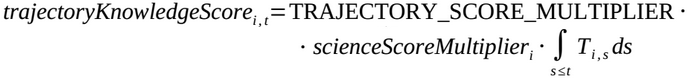where TRAJECTORY_SCORE_MULTIPLIER is a constant that represents the importance of trajectory information.

### Final score

Your final score for the test case will be: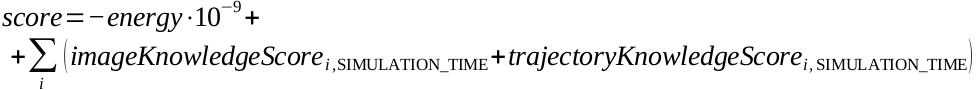where SIMULATION_TIME is the end of the simulation time period.

If you were not able to complete the simulation (due to time limit, memory limit, crash, invalid return value, etc.), then your score for the test case will be 0. If your score is negative, it is reset to 0. Your total score is the average of your scores on all test cases.

## Implementation

You will need to implement three methods, initialize, asteroidAppearance and nextCommand.

initialize will be called only once and before all other calls. It serves to provide your solution with information about the test case, including antenna positions, antenna parameters, etc. The complete list is:

• double[] antennaPositions, antenna ground position, formatted as: [X0, Y0, X1, Y1, ...]. The Z coordinate is 0 for all antennas.
• double peakGain, the peak gain for a single antenna
• double[] minDistanceGain, gain values generated for min distance between antennas
• double[] maxDistanceGain, gain values generated for max distance between antennas
The return value from initialize will be ignored. The initial directions of the antennas are straight up, i.e. towards (X, Y, Z) = (0, 0, 1). The length of minDistanceGain will always equal the length of maxDistanceGain. The number of antennas in the test case can be calculated by dividing the length of antennaPositions by two.

asteroidAppearance will be called whenever a new asteroid appears. It provides all information about the asteroid:

• int asteroidIndex, a unique index
• double scienceScoreMultiplier
• double reflectivityMultiplier
• double initialImageInformation
• double initialTrajectoryInformation
• double[] trajectory, formatted as: [Time0, X0, Y0, Z0, Time1, X1, Y1, Z1, ...]
The return value from asteroidAppearance will be ignored. double[] trajectory is ordered, i.e. Timen < Timen+1. The asteroid position between Timen and Timen+1 is (Xn, Yn, Zn).

nextCommand will be called repeatedly until the end of simulation. It provides the current time and you should return a string that specifies your command. It can be one of the two following formats:

• Change transmitting power:

<time> <antennaIndex> P <new transmit power>
• Relocate:

<time> <antennaIndex> R <new asteroid index>
Your command should satisfy:
• time ≥ current time
• 0 ≤ antennaIndex < number of antennas
• 0 ≤ new transmit power ≤ MAX_TRANSMITTING_POWER
• new asteroid index must have appeared

### Constants

Here is a list with all constants used in this problem:

• SPEED_OF_LIGHT = 299792458
• MAX_TRANSMITTING_POWER = 40000; 40 kW
• BACKGROUND_NOISE = 10-30
• SHIELDING_MULTIPLIER = 10-27
• RELOCATE_SPEED = π / (10 * 60); 10 min to relocate 180°
• RELOCATE_POWER = 5000; 5 kW
• SIMULATION_TIME = 7 * 24 * 60 * 60; 7 days
• T_MIN = 0.1
• CRITICAL_TRACKING_ANGLE = π / (180 * 60); 1 arc minute
• ANTENNA_SAFE_RADIUS = 11; 11 meters
• Q_LOST = (24 * 60 * 60) / log(2); half-life: 24h
• Q_TRAJECTORY = 6000
• Q_IMAGE = 1 000 000
• IMAGE_SCORE_MULTIPLIER = 30
• TRAJECTORY_SCORE_MULTIPLIER = 60 / SIMULATION_TIME

### Test case generation

Each test case is generated using the same algorithm, but with a different seed for the random number generator. The algorithm is described in this section.

First, the number of asteroids is chosen. It is chosen uniformly between 25 and 75, inclusive. A random subset with that many elements is then selected from this file. The first column is asteroid designation and the second column is asteroid absolute magnitude. Next, a starting day between year 2000 and year 2020, inclusive, is chosen uniformly at random. The geocentric positions for the asteroid subset over a week is then calculated using Minor Planet Ephemeris Service with 3 minutes intervals from the starting day. These geocentric positions are then converted so that they are relative to the antenna array origin. The antenna array origin at the starting day is located at latitude 28.524812° longitude 0°, and rotates one revolution around the Earth's axis in exactly 24 hours. Next, the number of antennas are chosen uniformly at random between 8 and 20, inclusive. A random subset with that many elements is then selected from this file that specifies the antenna ground positions. The reflectivityMultiplieri and scienceScoreMultiplieri for each asteroid i is calculated in the following way: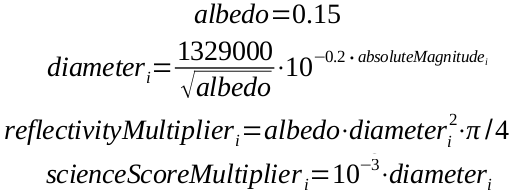The initialImageInformationi is chosen uniformly at random between 0 and 0.1. An initial start time is then chosen uniformly at random for each asteroid between -0.3 · SIMULATION_TIME and SIMULATION_TIME inclusive. The first time the asteroid appears is then calculated as max(0, initial start time). If the first time the asteroid appears is 0, initialTrajectoryInformationi is chosen uniformly at random between 0.2 and 4.0, inclusive. Otherwise, if the first time the asteroid appears is > 0, initialTrajectoryInformationi is chosen uniformly at random between 0.2 and 0.4, inclusive. The asteroid index is simply the index in the subset, between 0 and number of asteroids - 1, inclusive.

The values peakGain, minDistanceGain and maxDistanceGain are generated using a complex formula, but you can expect very similar characteristics in the system test cases.

### Tools

An offline tester/visualizer is available . You can use it to test/debug your solution locally.

100 extra test cases for you to play with offline is provided here .

You are encouraged to check the source code for exact implementation of the simulation and score calculation. Feel free to use the simulator code in your solution.

### Definition

 Class: AsteroidTracker Method: initialize Parameters: double[], double, double[], double[] Returns: int Method signature: int initialize(double[] antennaPositions, double peakGain, double[] minDistanceGain, double[] maxDistanceGain) Method: asteroidAppearance Parameters: int, double, double, double, double, double[] Returns: int Method signature: int asteroidAppearance(int asteroidIndex, double scienceScoreMultiplier, double reflectivityMultiplier, double initialImageInformation, double initialTrajectoryInformation, double[] trajectory) Method: nextCommand Parameters: double Returns: String Method signature: String nextCommand(double currentTime) (be sure your methods are public)

### Notes

-The time limit is 30 seconds (this includes only the time spent in your code). The memory limit is 1024 megabytes.
-Doppler effects are ignored in this contest. In reality, the duration of the emitted signal does not equal the duration of the received signal due to movement of the object, and the power of the signal is modified accordingly. Because this effect is ignored in this contest, there will be some small numerical artifacts as a result of the step incremental movements of the asteroids. One of these artifacts is that for some (really small) periods of time, it is pointless to transmit, as the period will be overwritten by the future signal. As asteroids are much slower than light, this effect has a negligible impact on the score.
-The trajectory knowledge score is integrated numerically using a linear approximation between events.
-There is no explicit code size limit. The implicit source code size limit is around 1 MB (it is not advisable to submit codes of size close to that or larger). Once your code is compiled, the binary size should not exceed 1 MB.
-The compilation time limit is 30 seconds. You can find information about compilers that we use and compilation options here.
-There are 10 example test cases and 100 full submission (provisional) test cases.

### Examples

0)

```Seed = 1
Number of antennas = 17
Number of asteroids = 47
peakGain = 941857.0
```
1)

```Seed = 2
Number of antennas = 14
Number of asteroids = 69
peakGain = 1194340.0
```
2)

```Seed = 3
Number of antennas = 11
Number of asteroids = 40
peakGain = 994527.0
```
3)

```Seed = 4
Number of antennas = 8
Number of asteroids = 62
peakGain = 1258740.0
```
4)

```Seed = 5
Number of antennas = 17
Number of asteroids = 33
peakGain = 1047910.0
```
5)

```Seed = 6
Number of antennas = 14
Number of asteroids = 56
peakGain = 1323890.0
```
6)

```Seed = 7
Number of antennas = 20
Number of asteroids = 64
peakGain = 1489580.0
```
7)

```Seed = 8
Number of antennas = 20
Number of asteroids = 55
peakGain = 1308430.0
```
8)

```Seed = 9
Number of antennas = 20
Number of asteroids = 46
peakGain = 1114670.0
```
9)

```Seed = 10
Number of antennas = 20
Number of asteroids = 37
peakGain = 1333600.0
```

This problem statement is the exclusive and proprietary property of TopCoder, Inc. Any unauthorized use or reproduction of this information without the prior written consent of TopCoder, Inc. is strictly prohibited. (c)2010, TopCoder, Inc. All rights reserved.